### 2.3.2.3 Isotropic Distribution Function

Eqns. (2.76) to (2.78) all contain a statistical average of a symmetric tensor (see Appendix A) of the form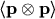which will be evaluated in this section. The distribution function can be decomposed into a symmetric and an anti-symmetric part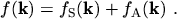(2.79)

In this section we assume that the symmetric part is isotropic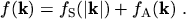(2.80)

This is a special case of the diffusion approximation [21, p.49] which will be explained in more detail for the MAXWELL distribution in Section 2.3.3.1 on page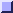. By using this assumption the statistical average of the tensor can be written as(2.81)

For symmetry reasons all elements outside the trace vanish. For instance, the element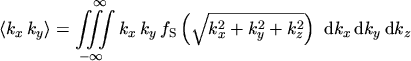(2.82)

evaluates to zero because of the integral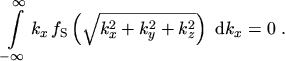(2.83)

Since the distribution function is assumed to be isotropic, the integrals determining the elements of the trace all evaluate to a common value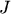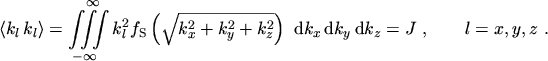(2.84)

The value ofcan be evaluated by the simple transformation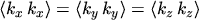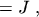(2.85)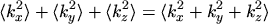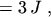(2.86)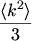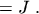(2.87)

Therefore, the statistical averages of the tensors are diagonal with all diagonal elements being equal: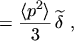(2.88)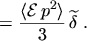(2.89)

By inserting eqns. (2.88) and (2.89) into eqns. (2.76) to (2.78) one gets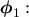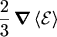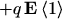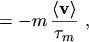(2.90)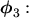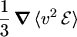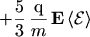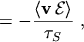(2.91)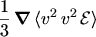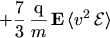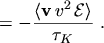(2.92)

Note that the divergences of the tensors simplify to gradients of scalars.

M. Gritsch: Numerical Modeling of Silicon-on-Insulator MOSFETs PDF